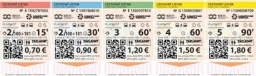# Tickets 18603

The cashier at the cinema sells tickets for 50 CZK and 40 CZK. He has sold 143 tickets and earned a total of CZK 6,340. How many tickets did he sell?

a =  62
b =  81

### Step-by-step explanation:

a+b = 143
50a + 40b = 6340

a+b = 143
50·a + 40·b = 6340

a+b = 143
50a+40b = 6340

Pivot: Row 1 ↔ Row 2
50a+40b = 6340
a+b = 143

Row 2 - 1/50 · Row 1 → Row 2
50a+40b = 6340
0.2b = 16.2

b = 16.2/0.2 = 81
a = 6340-40b/50 = 6340-40 · 81/50 = 62

a = 62
b = 81

Our linear equations calculator calculates it.Did you find an error or inaccuracy? Feel free to write us. Thank you!

Tips for related online calculators
Do you have a system of equations and looking for calculator system of linear equations?